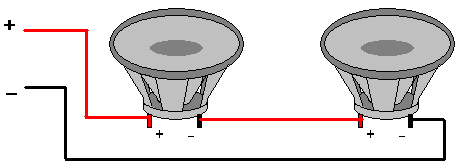## Series Wiring of LoudspeakersUsing two 8 ohm speakers the total resistance is given by the formula;

RT = R1 + R2

RT = 8 + 8

RT = 16 ohms

For loudspeakers in series;
Just add the values of all the resistances together;

Rt = R1 + R2 + R3.

## Parallel Wiring of LoudspeakersUsing two 8 ohm speakers the total resistance is given by the formula;

1/RT = 1/R1 + 1/R2

1/RT = 1/8 + 1/8 =2/8

Turn fraction upside down to get

RT = Rt = 8/2 = 4 ohms

From the previous Diagrams you will see that for two 8 Ohm speakers In parallel, the total resistance is half of the value of one speaker 4 Ohms.
For 2 16 Ohm speakers in parallel;

The total resistance is half of 16. And thus = 8 Ohms

If you use 3 speakers of equal value then total resistance will be a third of the resistance of one speaker.

3 x 8 Ohm speakers; Total R = 8/3 Ohms.

For different value speakers use the formula provided above.

For two speakers of different value in parallel;

1/Rt = I/16 + 1/8 = 1/16 + 2/16 = 3/16

Rt thus = 16/3 Ohms = 5.3 Ohms

For three speakers of different value in parallel;

using 16 Ohm 8 Ohm and 4 Ohm

1/Rtt = 1/R1 + 1/R2 + 1/R3

Rt = 1/16 + 1/8 + 1/4

as 2/16 = 1/8 and 4/16 = 1/4

RT= 1/16 + 2/16 + 4/16

1/Rt = 7/16

Rt = 16/7 = 2.3 Ohms

For speakers in parallel the total resistance is roughly half of the lowest resistance speaker.

Warning! You can blow your amplifier up by running it into too low a speaker resistance.